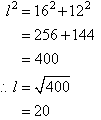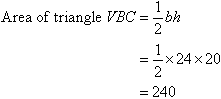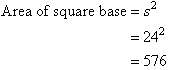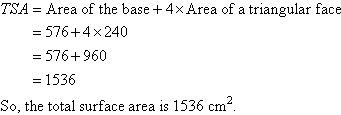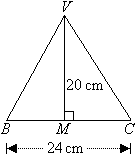Year 10 Interactive Maths - Second Edition

## Total Surface Area of a Pyramid

A pyramid is a three-dimensional figure made up of a base and triangular faces that meet at the vertex, V, which is also called the apex of the pyramid.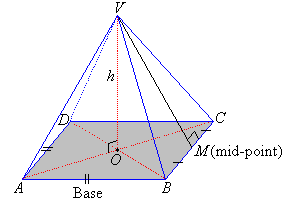The number of triangular faces depends on the number of sides of the base.  For example, a pyramid with a rectangular base has four triangular faces, whereas a pyramid with a hexagonal face is made up of six triangular faces, and so on.

The lower face ABCD is called the base and the perpendicular distance from the vertex, V, to the base at O is called the height of the pyramid.  The total surface area of a pyramid is the sum of the areas of its faces including its base.

###### Note:
• A square pyramid has four equal triangular faces and a square base.
• A pyramid does not have uniform (or congruent) cross-sections.

#### Example 30

Find the total surface area of a square pyramid with a perpendicular height of 16 cm and base edge of 24 cm.

##### Solution: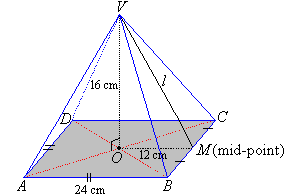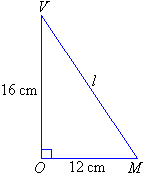By Pythagoras' Theorem from right-triangle VOM, we have